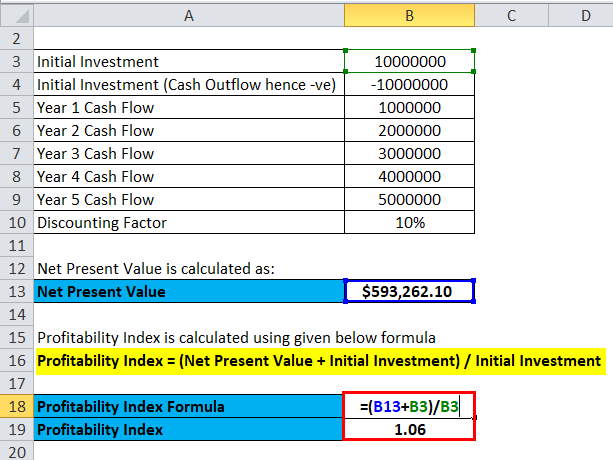# How To Calculate Profitability Index In Excel

As a business owner or investor, it’s essential to have a clear understanding of the profitability of your investments. Calculating profitability index (PI) is one of the most effective ways to measure the profitability of a potential investment. The PI helps you determine the value of an investment relative to the cost of the investment, taking into account the time value of money.

If you’re looking for a way to calculate PI quickly and accurately, you’re in the right place. In this article, we’ll show you how to calculate profitability index in Excel step-by-step. Whether you’re an experienced Excel user or just starting out, we’ll provide you with all the information you need to make informed investment decisions and maximize your returns. So, let’s dive in!## Calculating Profitability Index in Excel

Profitability index, also known as the benefit-cost ratio, is a popular tool used to measure the returns of a project against its costs. It is a measure of the return on investment (ROI) and is a very useful tool for determining the profitability of a project or investment. In this article, we will discuss how to calculate Profitability Index in Excel.

### What is Profitability Index?

Profitability index (PI) is a measure of the returns of a project or investment against its costs. It is commonly used in business decision making and finance to determine the profitability of a project or investment. The profitability index is a ratio of the present value of the expected returns of a project to the present value of its costs. The higher the profitability index, the more profitable the project or investment is.

### Calculating Profitability Index in Excel

Calculating Profitability Index in Excel is relatively simple. The formula for calculating Profitability Index is as follows: PI = (Present Value of Future Cash Flows) / (Initial Investment). To calculate Profitability Index, you will need to enter the present value of the future cash flows, the initial investment, and the discount rate (if applicable).

Once you have entered the data into the formula, the result should be the Profitability Index. To calculate the Profitability Index, you will need to use the NPV (net present value) and IRR (internal rate of return) functions. The NPV function will calculate the present value of the expected cash flows, and the IRR function will calculate the internal rate of return.

Once you have calculated the Profitability Index, you can use it to compare different investments or projects to determine which is more profitable. For example, if one project has a higher Profitability Index than another, then it is more likely to be the more profitable project.

Profitability index, also known as the benefit cost ratio, is an important tool used in financial analysis to measure the return on investment. It is important to understand how to calculate profitability index in Excel to make accurate financial decisions.

### What is the Formula for Profitability Index in Excel?

The formula for profitability index in Excel is simply the present value of all future cash flows divided by the initial cost of the investment. This formula can be expressed as PV/I, where PV is the present value of future cash flows and I is the initial investment. This calculation gives an indication of how much money is being earned relative to the initial investment.

### How do I Calculate Profitability Index in Excel?

To calculate the profitability index in Excel, the first step is to enter the present value of all future cash flows into a cell. This can be calculated using the present value formula, which takes the cash flows for each period and discounts them back to the present. Once the present value has been calculated, the next step is to enter the initial investment in a separate cell, and then divide the present value by the initial investment. This will give the profitability index.

### What is a Good Profitability Index?

A good profitability index is one that is higher than the cost of capital for the investment. Generally, a profitability index of 1 or higher is considered a good indicator of a profitable investment. If the profitability index is less than 1, then the investment is not likely to be profitable in the long run.

### How Can I Improve Profitability Index?

The profitability index can be improved by increasing the present value of the future cash flows and/or decreasing the initial investment. This can be done by increasing the expected cash flows, reducing the cost of capital, or by utilizing investment strategies such as tax deferment or leveraging.

### What are the Limitations of Profitability Index?

The profitability index is a useful tool for evaluating investments, however, it does have some limitations. The profitability index does not take into account the timing of cash flows, so it does not provide a complete picture of the potential return on investment. Additionally, the profitability index does not consider the risk associated with the investment, or any other external factors that could affect the return on investment.

In conclusion, mastering the art of calculating profitability index in Excel is a vital skill for any business owner or financial analyst. By applying the steps outlined in this guide, you can easily determine whether an investment project is worth pursuing or not. Remember, profitability index is a powerful tool that takes into account the time value of money and provides a clear picture of the potential return on investment.

So, whether you are looking to invest in a new venture or evaluate the performance of an existing one, using profitability index will help you make informed decisions. With practice and consistency, you can become an expert in Excel and use the software to analyze financial data with ease. So, go ahead and give it a try today, and watch your business grow and thrive.